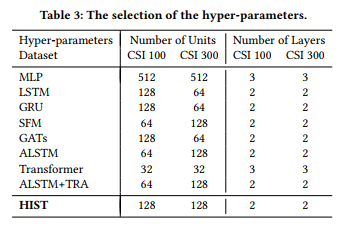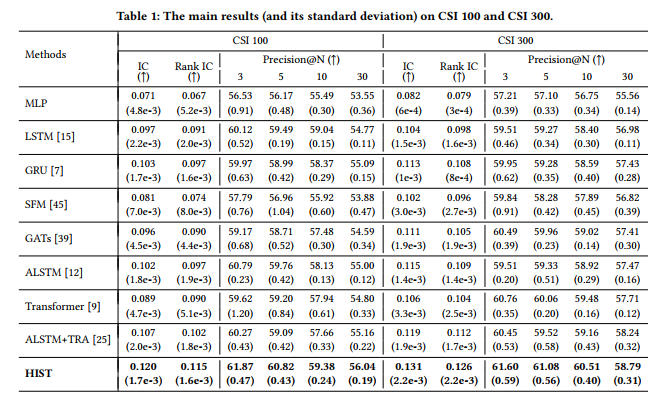## 基本介绍

• 股票所属板块所包含的共有的预定义信息（Predefined Concept）。
• 预定义中不包含的隐含信息（Hidden Concept）。
• 每支股票独有的特质信息。（Individual Concept）。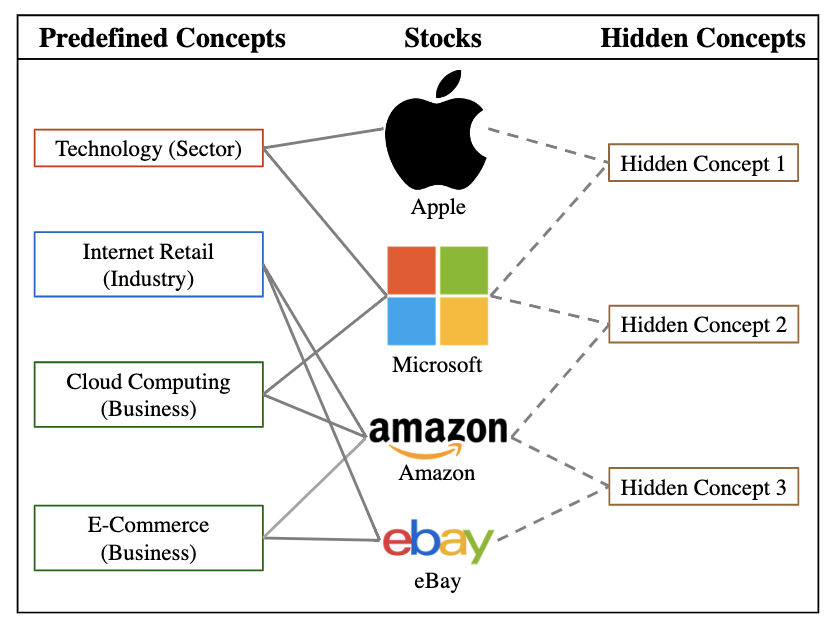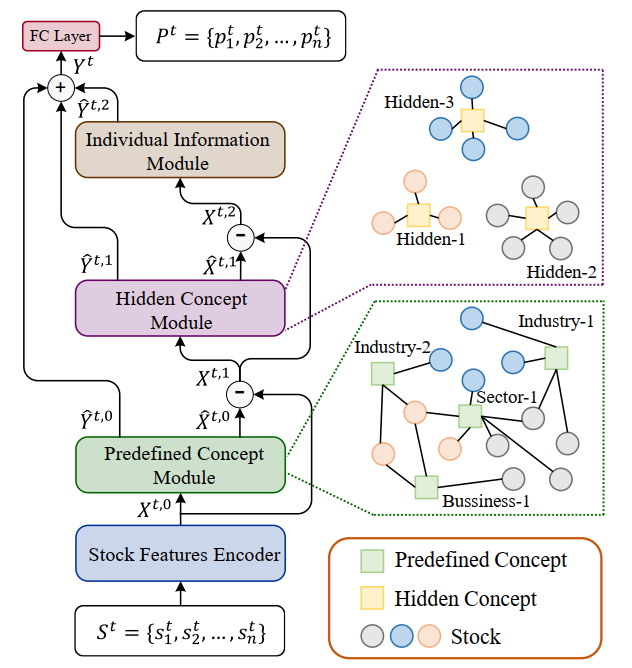## Stock Features Encoder

$x_i^t=GRU(\textbf s_i^t)$

## Predefined Concept Module

### 概念表征初始化

$\alpha_{k,i}^t = \frac{c_i^t}{\sum_{j\in N_k^t}c_j^t} \\ e_k^t=\sum_{j\in N_k^t}\alpha_{k,i}^tx_i^t$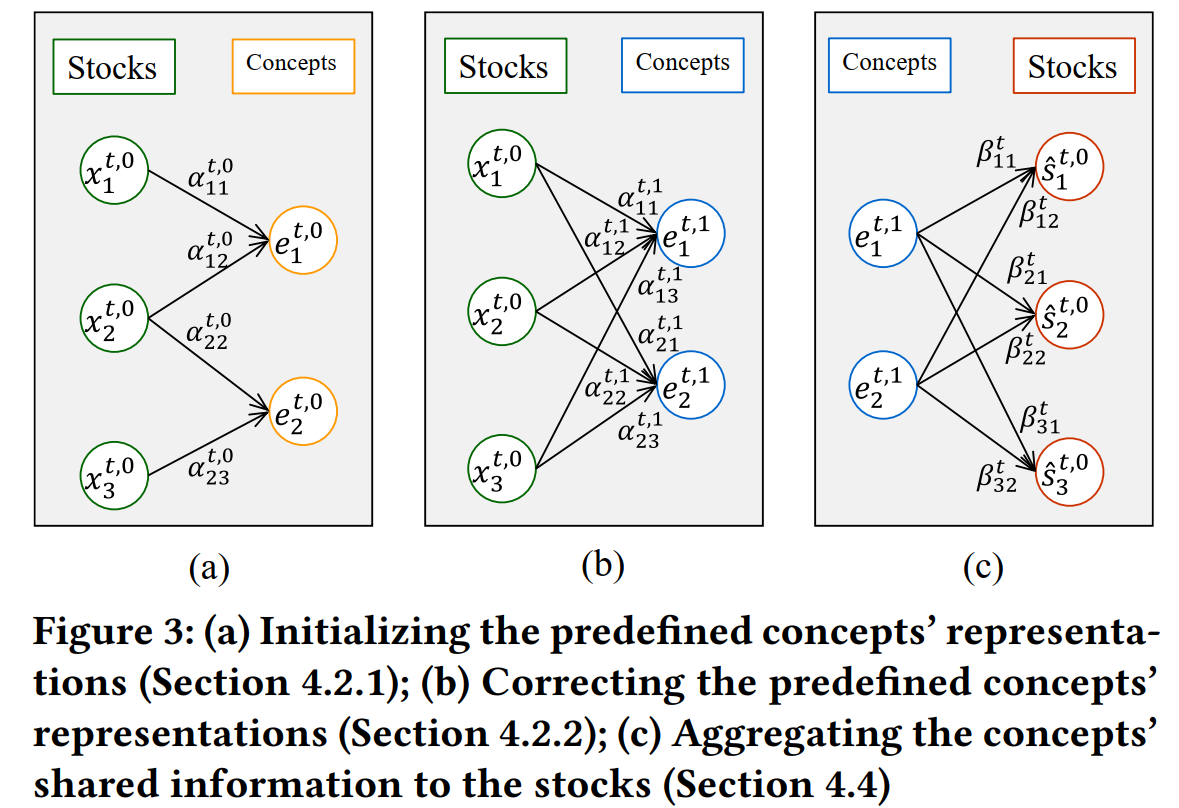### 概念表征处理

$\alpha_{k,i}^t = softmax(Cosine(x_i^t,e_k^t)) \\ e_k^t = LeakyReLU(W_e(\sum_{i\in S^t}\alpha_{k,i}^tx_i^t)+b_e)$

### 共有信息剥离

$\beta_{k,i}^t = softmax(Cosine(x_i^t,e_k^t)) \\ \hat s_i^t = LeakyReLU(W_s(\sum_{k\in K^t}\beta_{k,i}^te_k^t)+b_s)$

$\hat x_i^{t,0} = LeakyReLU(W_b\hat s_i^t+b_b) \\ \hat y_i^{t,0} = LeakyReLU(W_f\hat s_i^t+b_f)$## Hidden concept module

$\gamma_{k,i}^t=Cosine(x_i^{t,1}, u_i^{t,0})$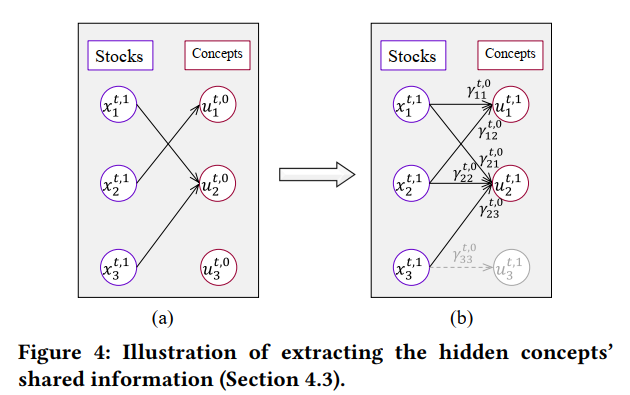$u_k^{t,1}=LeakyRelLU(W_u(\sum_{i \in N_k^t}\gamma_{k,i}^tx_i^{t,1})+b_u)$

## Individual information module

$\hat y_i^{t,2} = LeakyReLU(W_{f2}\hat x_i^{t,2}+b_{f2})$

## All Module

$p_i^t=W_py_i^t+b_p=W_p(\hat y_i^{t,0}+\hat y_i^{t,1}+\hat y_i^{t,2})+b_p$

$L=\sum_{t\in T}MSE(p^t,d^t)=\sum_{t\in T}\sum_{i \in S^t}\frac{(p^t-d^t)^2}{|S^t|} \\$

## 原文结论